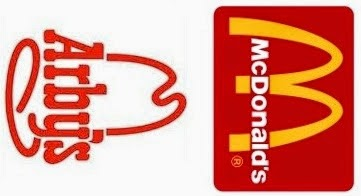## Wednesday, July 30, 2014

### 66 | French Fries, McDonald's and the "13 Colonies"

French Fries are something that seemed to be misnamed.  After all, they weren't created in France, but instead, Belgium, one of the world's eight "33" countries.
• Belgium = 2+5+3+7+9+3+4 = 33
The numerology of the world "thirty-three" has a connection to "french fries".  Let us examine, piece by piece.
• Thirty-Three = 2+8+9+9+2+7+2+8+9+5+5 = 66
Remember that number 66, it is an important one in more ways than you would believe.
• French Fries = 6+9+5+5+3+8+6+9+9+5+1 = 66
When the name is reduced with the Standard Method, it also produces a curious result.
• French = 6+18+5+14+3+8 = 54
• Fries = 6+18+9+5+19 = 57
• French Fries = 54+57 = 111
• Think about the number 111 in light of a box of slender, skinny french fries
Each year "McDonald's" sells the most french fries in the world.
• McDonald = 13+3+4+15+14+1+12+4 = 66
McDonald's is home of the "happy" meal.
• Happy = 8+1+16+16+25 = 66
Recall how "33" and "66" connect.  In Jewish Gematria, the abbreviation "Mc" is equal to "33" because M = 30 and C = 3.
• Mc = 30+3 = 33
• Jewish Gematria calculation
With regards to the number 66, it is central in the establishment of the first 13 colonies.  Please read this post to see how the sum of the first 13 colonies is "66"; also the first highway built across the nation, running from Chicago, home of the 60606 area code, to Los Angeles and the Pacific, was Route 66- but that's not all, so please read this blog!Can you see the 13?